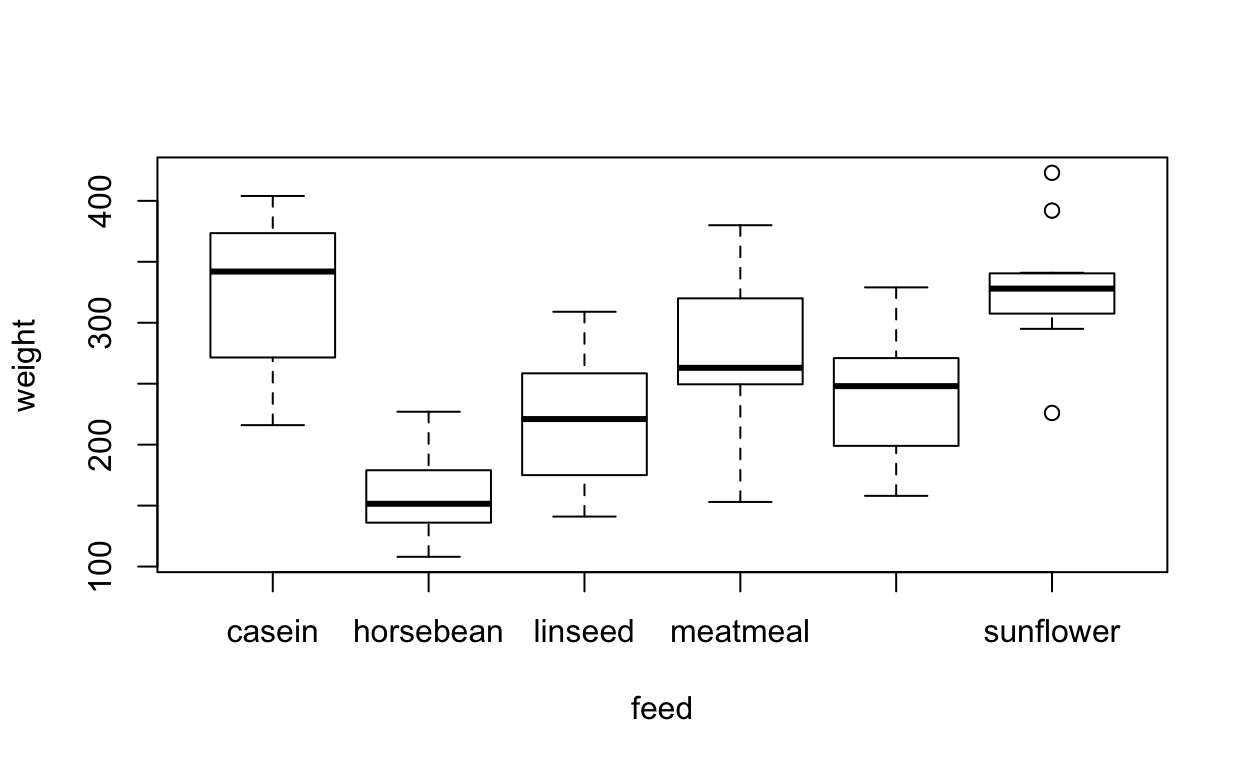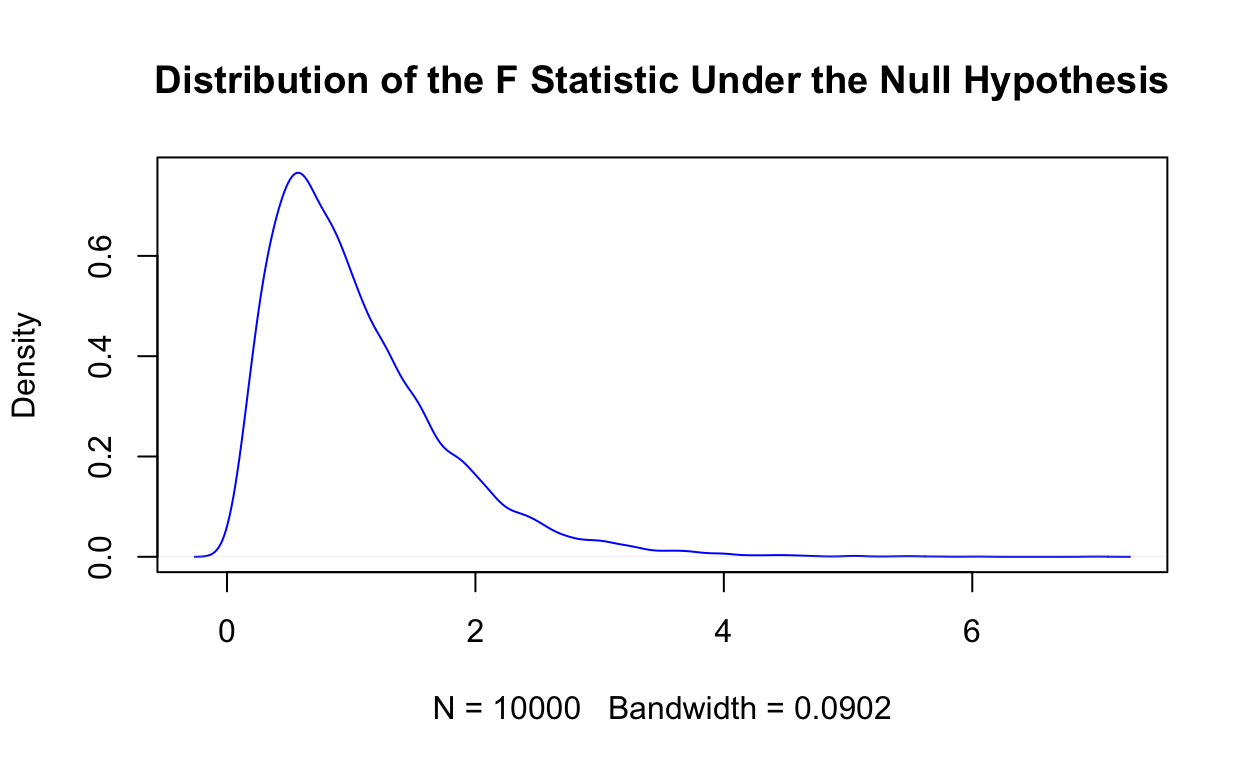# Precept 5

ANOVA Calculations - Step By Step

Federico H. Tiberti pol346.com (Princeton University, Department of Politics)princeton.edu/politics
2020-03-06

## Introduction

In this document we will perform an ANOVA analysis to test a hypothesis of difference across multiple samples. To do so, we will rely on a built-in dataset in R, Chicken Weights. This dataset contains data on chicken weights and feed type for 71 observations. First, we load the packages and the data:


library(janitor)
library(dplyr)
library(summarytools)
library(ggplot2)
library(kableExtra)

chicken <- chickwts
head(chicken)

weight      feed
1    179 horsebean
2    160 horsebean
3    136 horsebean
4    227 horsebean
5    217 horsebean
6    168 horsebean
There are six different categories of feed type:

tabyl(chickenfeed, sort=TRUE, show_na = FALSE) %>% knitr::kable(col.names = c("Feed", "n", "%"), align = "c", digits=2) Feed n % casein 12 0.17 horsebean 10 0.14 linseed 12 0.17 meatmeal 11 0.15 soybean 14 0.20 sunflower 12 0.17 A simple boxplot gives us an idea about the distribution of weight by feed type.  plot(weight~feed, data=chicken)The research question we can address with this data is whether feed type has an effect on chicken weight. If it had an effect, we should observe significant difference in weight across different feed types. To try and answer that question, an ANOVA approach is well suited, as it allows us to compare multiple samples without collapsing the data to a pairwise comparison. Our null hypothesis is that the mean weight across all six feed types is the same: $\mu_{casein} = \mu_{horsebean} = \mu_{linseed} = \mu_{meatmeal} = \mu_{soybean} = \mu_{sunflower}$ Conceptually, the ANOVA test evaluates how much of the explanatory power we gain from fitting more means within the sample (with respect to just fitting a grand mean). In other words, the F-test determines whether the variability between group means is larger than the variability of the observations within the groups. If that ratio (F-statistic) is sufficiently large, we can reject the null hypothesis that all means are equal. To calculate this ratio, we need to calculate the extra sum of square residuals, ie the difference between total sum of squares when fitting the reduced (equal means) model versus the full (separate means) model. The code below computes those residuals for each observation.  chicken <- chicken %>% mutate(mean_weight = mean(weight)) %>% # creates the grand mean group_by(feed) %>% #groups observations by feed category mutate(feed_means = mean(weight)) %>% # creates means by group ungroup() %>% mutate(residual_within = (weight-feed_means)^2, # calculates square residual from group mean residual_between = (feed_means-mean_weight)^2, # calculates the individual "extra square residual" residual_total = (weight-mean_weight)^2) # calculates square residual from grand mean The extra sum of square residuals is calculated below:  total_ss <- sum(chickenresidual_total)

within_ss <- sum(chicken$residual_within) extra_ss <- total_ss - within_ss In a similar way, we get the extra degrees of freedom as the difference in dfs between the full and reduced models:  total_df <- nrow(chicken)-1 within_df <- nrow(chicken) - length(unique(chicken$feed))

extra_df <- total_df - within_df

Conveniently, having already calculated the sum of square residuals and the degrees of freedom of the full model allows us to easily compute the pooled estimate of the variance from the full model:


pooled_variance <- within_ss / within_df

So now it’s just a matter of calculating the mean square (dividing the extra sum of squares by the extra degrees of freedom) and scaling it by the pooled estimate of the variance to get to the F-statistic:


mean_square <- extra_ss / extra_df
mean_square

 46225.83

f_stat <- mean_square / pooled_variance
f_stat

 15.3648

We can see how extreme such a value is by plotting the distribution of the F-statistic under the null. In this case, the null distribution would be an F distribution with 5 degrees of freedom in the numerator (the “extra degrees of freedom”) and 65 degrees of freedom in the denominator (the “full model” degrees of freedom). Below, we plot 10,000 random draws from such distribution:


rf(10000, df1 = extra_df, df2 = within_df) %>%
density() %>%
plot(main= "Distribution of the F Statistic Under the Null Hypothesis", col = "blue")We can also use that F distribution to calculate the p-value of our F-statistic:


# NOTE: setting lower.tail = FALSE gives us right tail
pf(f_stat, 5, 65, lower.tail = FALSE) 

 5.93642e-10

# repeat with high "penalty" for scientific notation
options(scipen = 10)
pf(f_stat, 5, 65, lower.tail = FALSE) 

 0.000000000593642

The p-value es extremely low, therefore we can reject the null hypothesis that all the means are equal. To check the robustness of our manual calculation, we can use the built-in aov() function:


aov(weight ~ feed, data = chicken) %>%
summary()

Df Sum Sq Mean Sq F value         Pr(>F)
feed         5 231129   46226   15.37 0.000000000594 ***
Residuals   65 195556    3009
---
Signif. codes:  0 '***' 0.001 '**' 0.01 '*' 0.05 '.' 0.1 ' ' 1

Which yields the same F-statistic and p-value of our manual calculation.